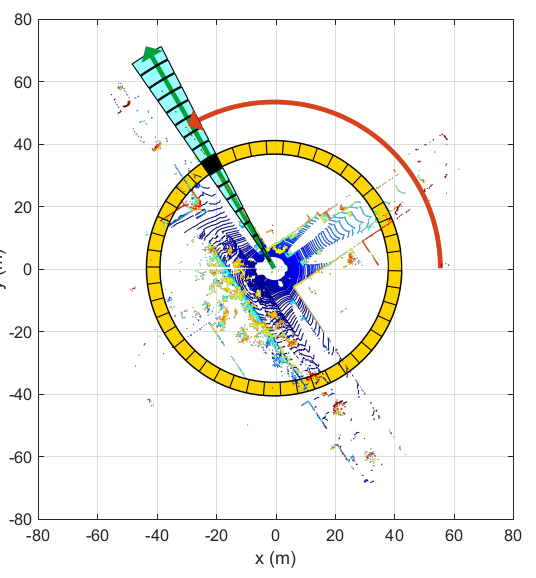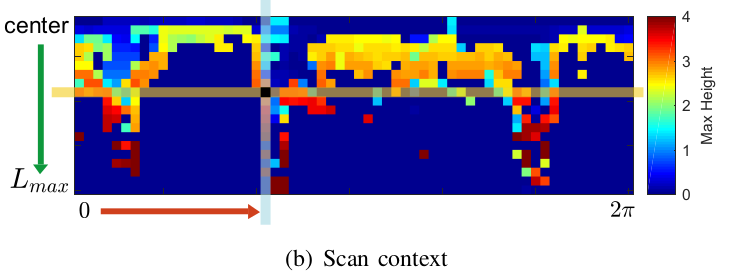## 介绍#

• 高效的数据编码方式。这种编码对点云的密度和法线具有不变性。
• 保存点云的内部结构。
• 有效的两相匹配算法。
• 与其他最先进的空间描述符进行了彻底的验证。## Scan Context#

$\mathcal{P} = \bigcup_{i\in[N_r],\space j\in[N_s]}P_{ij}$

$\phi=\mathcal{P}_{ij}\rightarrow\mathbb{R}$

$\phi(\mathcal{P_{ij}})=\max_{\mathbf{p}\in\mathcal{P}_{ij}}{z}(\mathbf{p})$

$I=(a_{ij})\in \mathbb{R}^{N_r\times N_s},a_{ij}=\phi(\mathcal{P_{ij}})$

## Scan Contexts之间的相似分数#

$d(I^q, I^c)=\frac{1}{N_s}\sum^{N_s}_{j=1}\left(1-\frac{c^q_j\cdot c^c_j}{\left \| c^q_j \right \| \left \| c^c_j \right \| }\right)$

\begin{align} D(I^q,I^c)&=\min_{n\in[N_s]}d(I^q,I^c_n), \\ n^*&=\text{argmin}_{n\in[N_s]}d(I^q,I^c_n) \end{align}

## 两相搜索算法#

$\mathbf{k}=(\psi(r_1),\dots,\psi(r_{N_r})), \space \text{where} \space \psi: r_i\rightarrow \mathbb{R}$

$\psi(r_i)=\frac{\|r_i\|_0}{N_s}$

$c^*=\text{argmin}_{c_k\in C} D(I^q, I^{c_k}), \space \text{s.t} \space D < \tau$